# Problem 1: Between-group statistics

Scientists are studying whether certain genetic modifications reduce the number of eggs a fruit fly, Drosophila, lays per day. Two types of flies, labeled RS and SS in the table (see attached files) have been bred to be Resistant to a pesticide or Susceptible to it, and the NS is the control group.

As a beginning statistician you were tasked with analyzing the data in several different ways. The instructions are below.

Read the data into an R table:

flyTable <- read.csv("PreExamExcerciseBetweenSs.csv")
1. Report descriptive statistics for each of the 3 groups: mean, median and box and whiskers plot. Indicate if there are any outliers that one could consider removing before analysis.

# Means of Groups
meansP1 <- data.frame(RS= mean(flyTable$Resistant.RS), SS= mean(flyTable$Susceptible.SS),
NS = mean(flyTable$Nonselected.NS)) # Medians of Groups mediansP1 <- data.frame(RS= median(flyTable$Resistant.RS),
SS= median(flyTable$Susceptible.SS), NS = median(flyTable$Nonselected.NS))

#Box and whiskers plots
outlierRS <- which(flyTable$Resistant.RS == boxplot(flyTable$Resistant.RS,
xlab = "group: Resistant.RS", ylab = "eggs laid")$out)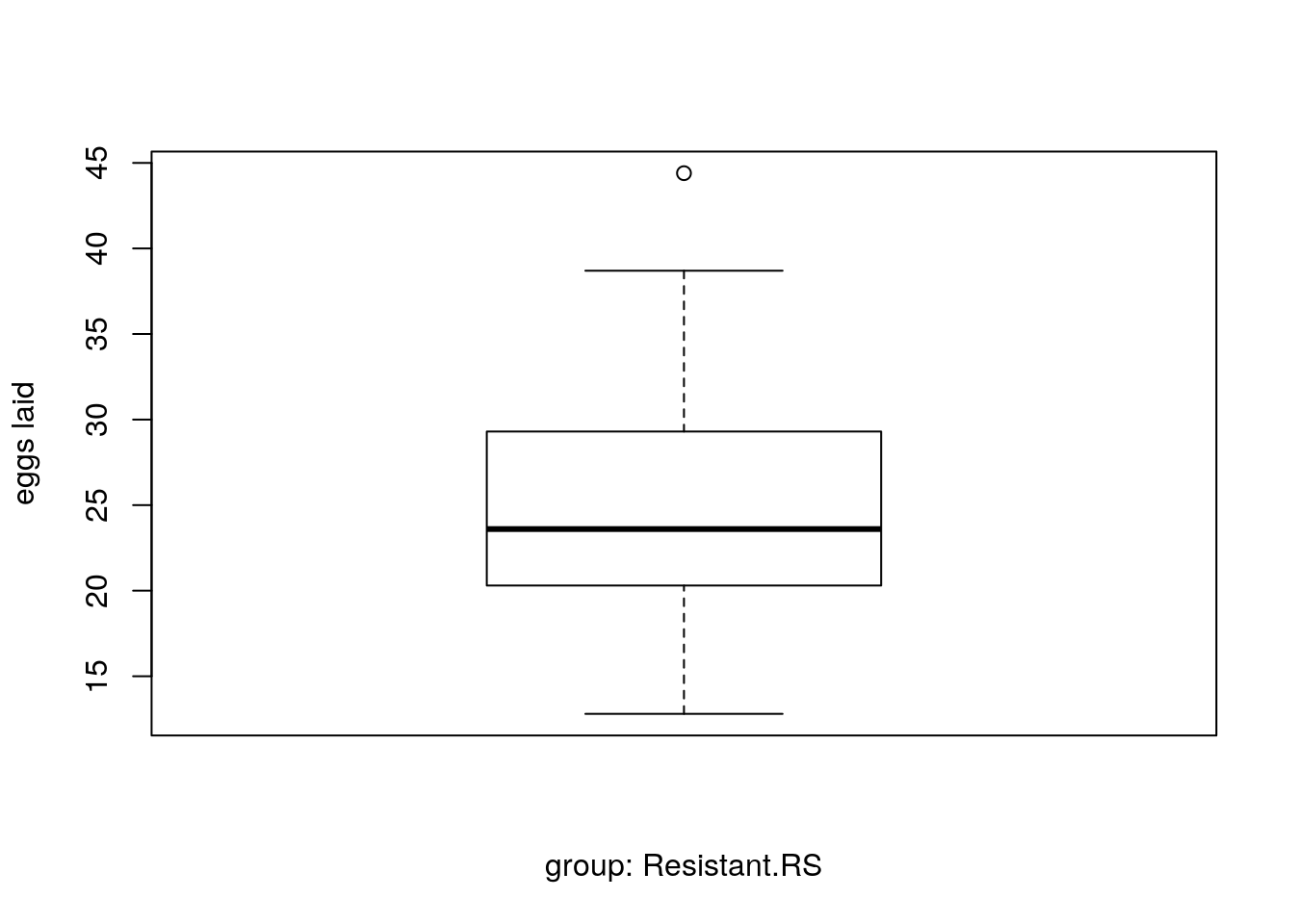outlierSS <- which(flyTable$Susceptible.SS ==
boxplot(flyTable$Susceptible.SS, xlab = "group: Susceptible.SS", ylab = "eggs laid")$out)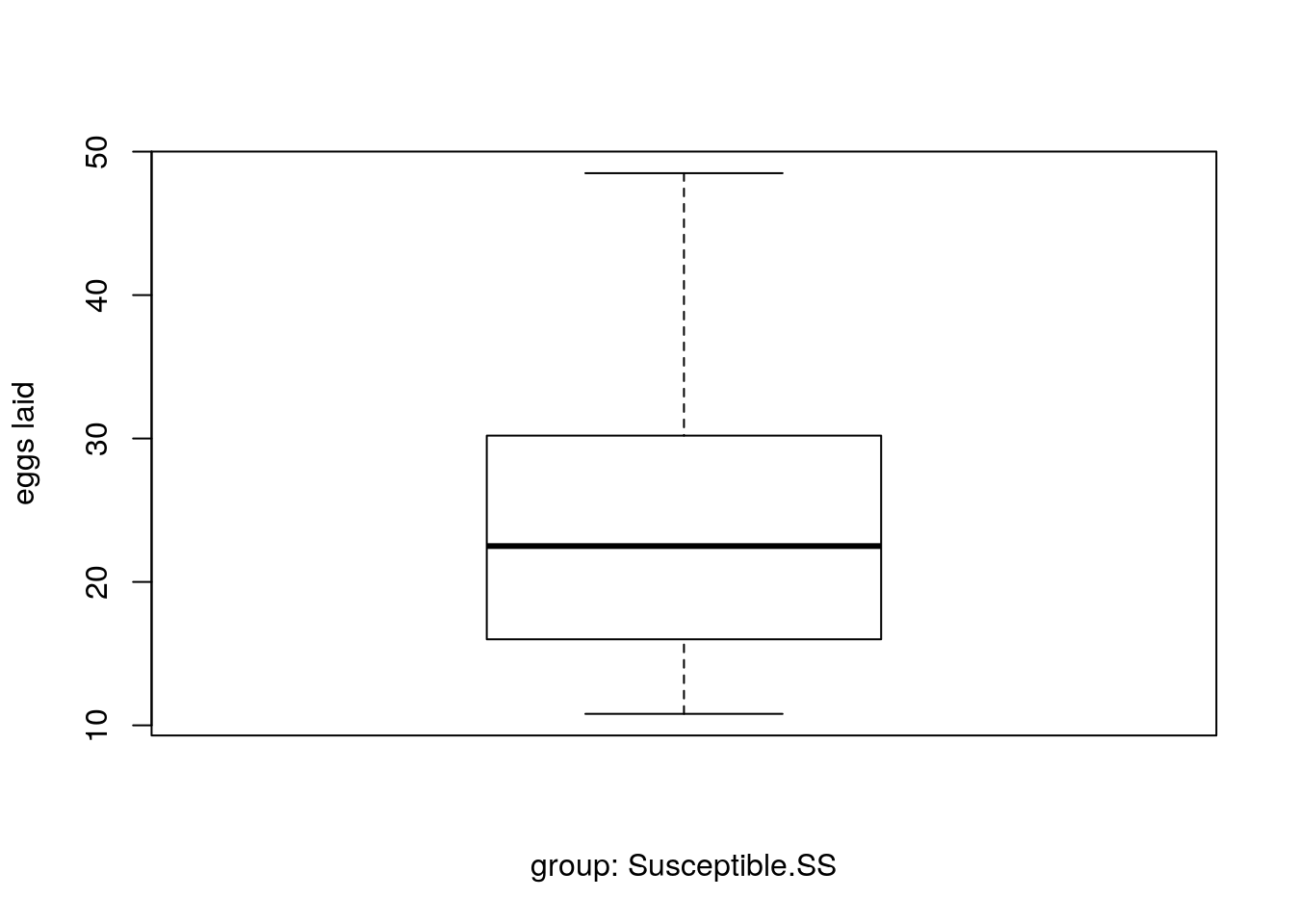outlierNS <- which(flyTable$Nonselected.NS == boxplot(flyTable$Nonselected.NS,
xlab = "group: Susceptible.NS", ylab = "eggs laid")$out)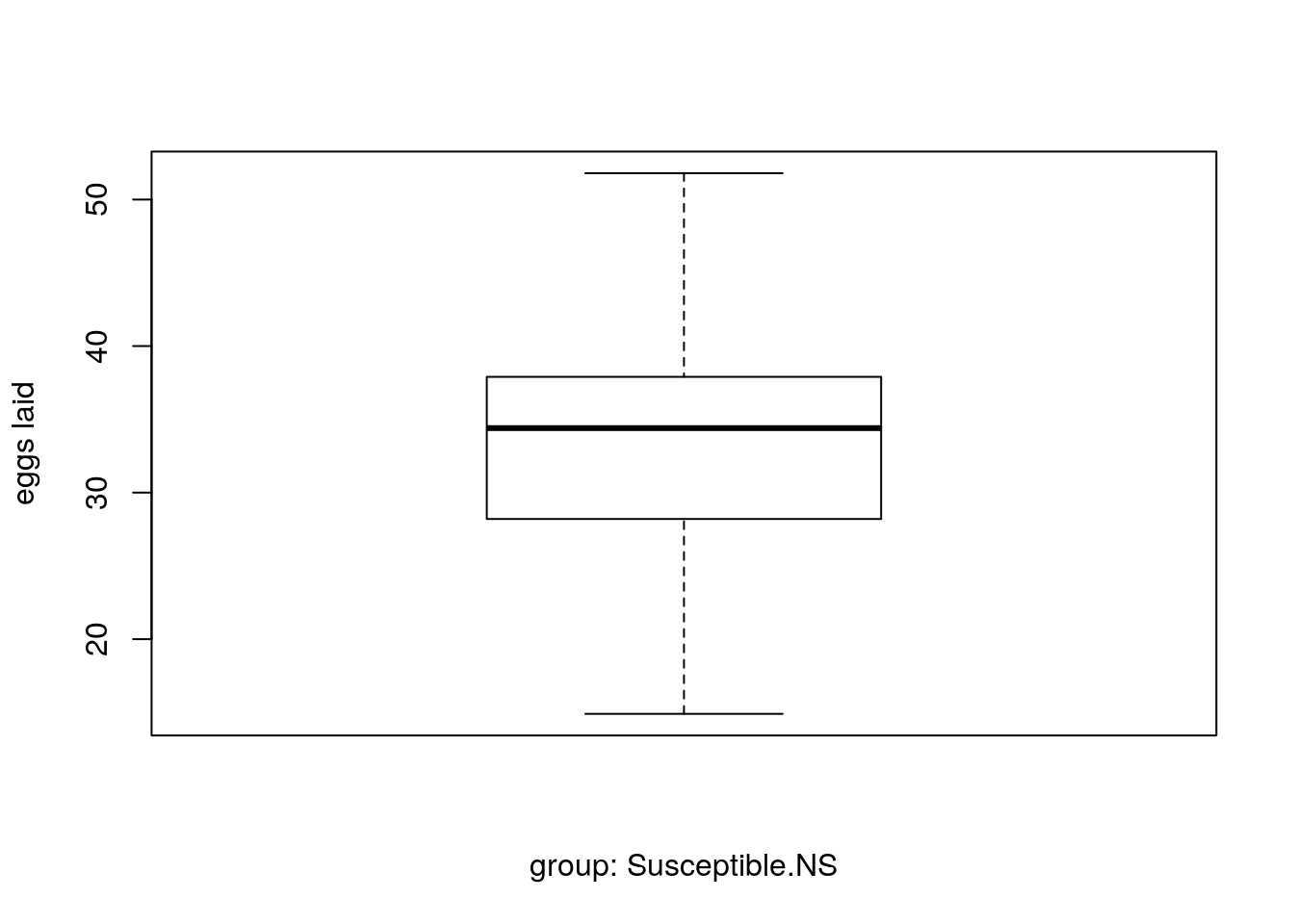print(paste("Number of Outliers of NS = ", length(outlierNS)), quote = FALSE) ##  Number of Outliers of NS = 0 print(paste("Number of Outliers of RS = ", length(outlierRS)), quote = FALSE) ##  Number of Outliers of RS = 1 print(paste("Number of Outliers of SS = ", length(outlierSS)), quote = FALSE) ##  Number of Outliers of SS = 0 2. Conduct the 3 pair-wise between-group T-tests between the groups and report which differ from the other. 1. State the null hypothesis and the alternative hypothesis for each test. • For Modified groups: Null: There is no significant difference in the number of leggs laid between group RS and group SS. Alternative: There is a significant difference in the number of eggs laid between group RS and SS. • For Comparison with control Group Null: There is no significant reduction in the number of laid eggs between group RS/SS in comparison to the contril group NS. (Two seperate tests) Alternative: There is a significant reduction in the number of laid eggs between group RS/SS in comparison to the contril group NS. (Two seperate tests) 2. How many degrees of freedom do each of these tests have? 48, because two groups of 25 and minus the number of groups. 3. Given that you are conducting 3 T-tests and want to control the overall Type-I error at alpha=.05, the statistical reliability of each test will need to be below .05/3 (<.0166). Use T-Tables or R’s qt function to look up the correct critical T value to evaluate which of the tests are statistically significant #t-test for RS and SS gorups t_RS_SS <- t.test(flyTable$Resistant.RS, flyTable$Susceptible.SS, alternative = "two.sided") t_RS_NS <- t.test(flyTable$Resistant.RS, flyTable$Nonselected.NS, alternative = "less") t_SS_NS <- t.test(flyTable$Susceptible.SS, flyTable$Nonselected.NS, alternative = "less") #calculate critical t-value for two sided modified groups t-test alpha = 0.05/3 tc_l_P1 = qt(alpha/2, df=48) # -2.48 tc_u_P1 = qt(alpha/2, df=48, lower.tail=FALSE) # 2.48 #calculate critical t-value for two other tests. tc_P1 <- qt(0.05/3, df = 48) # RS <-> SS if (t_RS_SS[["statistic"]][["t"]] > tc_u_P1 | t_RS_SS[["statistic"]][["t"]] < tc_l_P1) { print("RS-SS: reject H0")} else { print("RS-SS: don't reject H0")} ##  "RS-SS: don't reject H0" # RS <-> NS if (t_RS_NS[["statistic"]][["t"]] < tc_P1) { print("RS-NS: reject H0")} else { print("RS-NS: don't reject H0")} ##  "RS-NS: reject H0" # SS <-> NS if (t_SS_NS[["statistic"]][["t"]] < tc_P1) { print("SS-NS: reject H0")} else { print("SS-NS: don't reject H0")} ##  "SS-NS: reject H0" 1. Report effect size for each paired test as well as the confidence interval for the difference library(effsize) #calculate effect sizes # RS <-> SS effsizeRSSS <- cohen.d(flyTable$Resistant.RS, flyTable$Susceptible.SS) print.effsize(effsizeRSSS) ## ## Cohen's d ## ## d estimate: 0.1844334 (negligible) ## 95 percent confidence interval: ## lower upper ## -0.3854677 0.7543346 # SS <-> NS effsizeSSNS <- cohen.d(flyTable$Susceptible.SS, flyTable$Nonselected.NS) print.effsize(effsizeSSNS) ## ## Cohen's d ## ## d estimate: -0.9928783 (large) ## 95 percent confidence interval: ## lower upper ## -1.5955930 -0.3901637 # RS <-> NS effsizeRSNS <- cohen.d(flyTable$Resistant.RS, flyTable$Nonselected.NS) print.effsize(effsizeRSNS) ## ## Cohen's d ## ## d estimate: -0.9156189 (large) ## 95 percent confidence interval: ## lower upper ## -1.5133681 -0.3178697 3. Approach this problem using an ANOVA 1. What are the null and alternative hypotheses for the ANOVA test? • Null: There is no difference between the different three groups in terms of the number of the eggs laid. • Alternative: There is at least one significant difference in the number of eggs laid among at least one of the paired groups. 1. You have 3 groups with 25 ‘subjects’ each. What will be the degrees of freedom of the Numerator and Denominator terms in the F test? What would be the critical value for these degrees of freedom for alpha=.05? # degrees of freedom for Numerator (n of groups-1) dfNum <- nrow(t(flyTable))-1 # degrees of freedom for denominator (total n of samples - n of groups) dfDen <- length(t(flyTable))-nrow(t(flyTable)) #define critical value cv_fP1 <- qf(.95, dfNum, dfDen) 1. What does the F distribution with these degrees of freedom reflect? (hint: does it pertain to H0 or H1)? The F distribution shows the probability of seeing significant differences in the variances of 3 groups, assuming that there is no systematic difference between the conditions here groups. It thus pertains to H0. degrees of freedom affect critical value. If df are larger, the critical value will be smaller. 2. Calculate the ANOVA with R by computing s^2(W) and s^2(B) (by first calculating SSw and SSb) #calculate variance within groups SSw1 <- sum((flyTable$Resistant.RS - mean(flyTable$Resistant.RS))^2) SSw2 <- sum((flyTable$Susceptible.SS - mean(flyTable$Susceptible.SS))^2) SSw3 <- sum((flyTable$Nonselected.NS - mean(flyTable$Nonselected.NS))^2) SSWithin <- (SSw1 + SSw2 + SSw3) VarWithin <- SSWithin / (24 + 24 + 24) #calculate variance between SSBetween <- (sum(flyTable$Resistant.RS)^2)/25 +
(sum(flyTable$Susceptible.SS)^2)/25 + (sum(flyTable$Nonselected.NS)^2)/25 -
((sum(flyTable$Resistant.RS, flyTable$Susceptible.SS,
flyTable$Nonselected.NS))^2)/75 VarBetween <- SSBetween/2 #calculate F score Fobt <- VarBetween/VarWithin #Fobt=7.823 > cv_fP1(qf)=3.12, H0 can be rejected #now calculate ANOVA with R. #Stack the data table stacked_P1 <- stack(flyTable) 1. Use these to present an ANOVA table for the results. #ANOVA simp_Anova_1 <- aov(values ~ ind, data=stacked_P1) summary(simp_Anova_1) ## Df Sum Sq Mean Sq F value Pr(>F) ## ind 2 1223 611.6 7.823 0.000842 *** ## Residuals 72 5629 78.2 ## --- ## Signif. codes: 0 '***' 0.001 '**' 0.01 '*' 0.05 '.' 0.1 ' ' 1 1. Report Eta-squared Effect size #calculate effect size SSbetw/SStotal (SStotal=SSbetw+SSwith) etasq <- SSBetween/(SSWithin+SSBetween) #=0.178 >0.14, large effect size print(paste("Etha = ", etasq)) ##  "Etha = 0.178519261245391" 1. Use Tukey’s HSD to report which of the group-pairs significantly differed from each other tukey <- TukeyHSD(simp_Anova_1) tukey ## Tukey multiple comparisons of means ## 95% family-wise confidence level ## ## Fit: aov(formula = values ~ ind, data = stacked_P1) ## ##$ind
##                                 diff       lwr       upr     p adj
## Susceptible.SS-Resistant.RS   -1.628 -7.612719  4.356719 0.7924054
## Nonselected.NS-Resistant.RS    7.636  1.651281 13.620719 0.0087934
## Nonselected.NS-Susceptible.SS  9.264  3.279281 15.248719 0.0011874

# Problem 2: Paired T-tests and Repeated measures analyses

Determining the correct knee-joint angle for biking is important for comfortable biking. A researcher examined how setting this angle to different degrees impacted biking performance. The same 10 people were repeatedly measured over a number of weeks on a stationary bicycle with the knee-joint angle fixed at difference degrees. The Dependent variable was the distance biked until exhaustion.

The data are in the KneeBiking.csv file

kneeTable <- read.csv("KneeBiking.csv")
1. Report descriptive statistics for each of the 3 conditions : mean, median and box and whiskers plot.

#calculate means for 3 conditions
mean50 <- mean(kneeTable$X50deg) mean70 <- mean(kneeTable$X70deg)
mean90 <- mean(kneeTable$X90deg) #calculate meadians median50 <- median(kneeTable$X50deg)
median70 <- median(kneeTable$X70deg) median90 <- median(kneeTable$X90deg)

#plot box with whiskers
boxplot(kneeTable$X50deg, xlab = "Group: 50° knee angle", ylab = "Distance Cycled")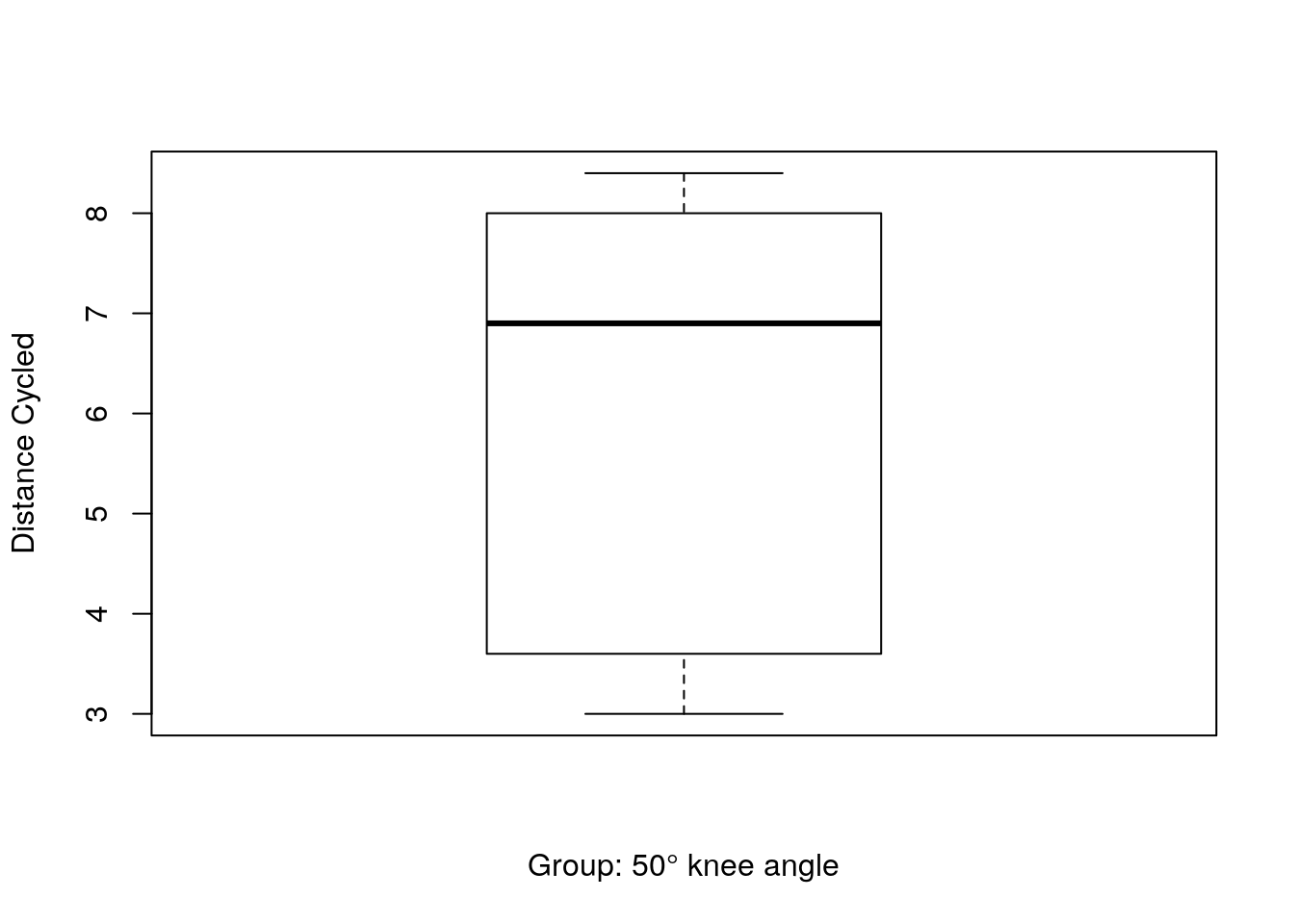boxplot(kneeTable$X70deg,
xlab = "Group: 70° knee angle", ylab = "Distance Cycled")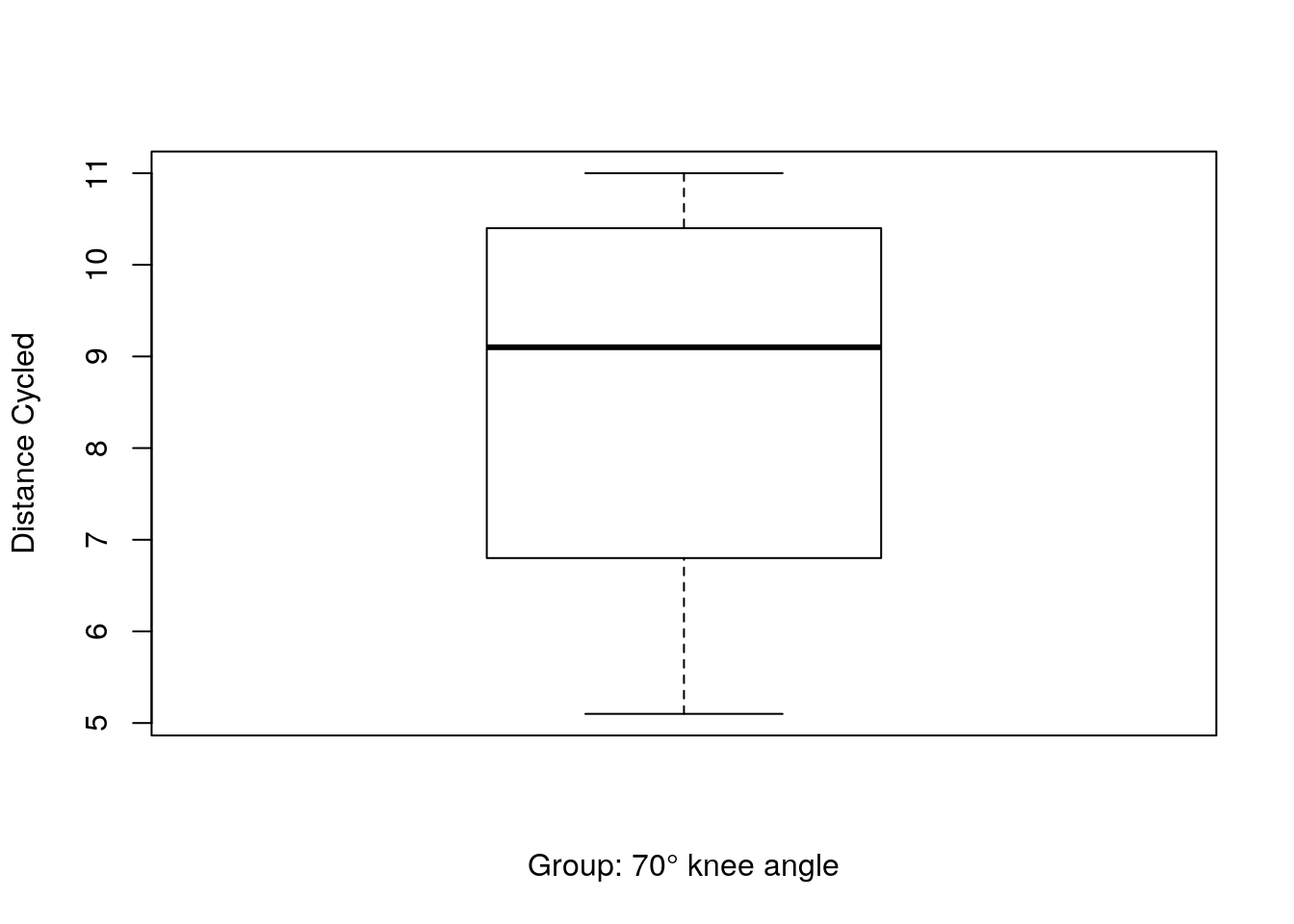boxplot(kneeTable$X90deg, xlab = "Group: 90° knee angle", ylab = "Distance Cycled")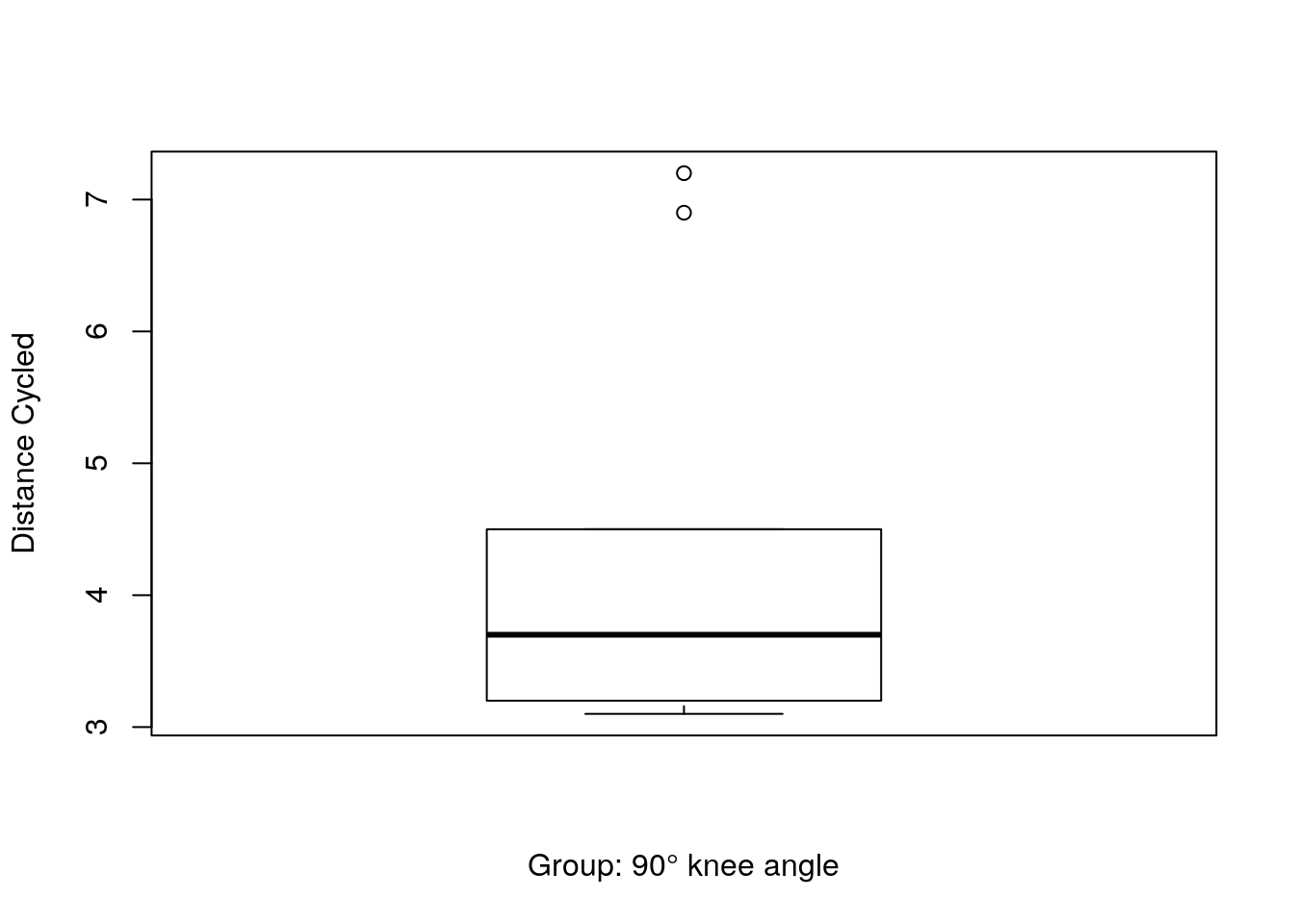2. Conduct the 3 pair-wise paired-samples T-tests and report which conditions differ from the other. 1. State the null hypothesis and the alternative hypothesis for each test. • 50 : 70 Null: There is no significant difference of ‘distance cycled’ between the two angle conditions 50° and 70° Alternative: There is a significant difference of distance cycled between the two angle conditions 50° and 70° • 50 : 90 Null: There is no significant difference of distance cycled between the two angle conditions 50° and 90° Alternative: There is a significant difference of distance cycled between the two angle conditions 50° and 90° • 70 : 90 Null: There is no significant difference of distance cycled between the two angle conditions 70° and 90° Alternative: There is a significant difference of distance cycled between the two angle conditions 70° and 90° 1. How many degrees of freedom do each of these tests have? The degree of the freedom is 9. Each trial consists of 10 fixed cases which makes it 9. 2. Given that you are conducting 3 Tests and want to control the overall Type-I error at alpha=.05 considering all 3 tests, the statistical reliability of each test will need to be below .05/3 (<.0166). Use T-Tables or R’s qt function to look up the correct critical T value to evaluate which of the tests are statistically significant. #t test for 50 and 70 degree ttest5070 <- t.test(kneeTable$X50deg, kneeTable$X70deg, paired = TRUE, alternative = "two.sided") #t test for 70 and 90 ttest7090 <- t.test(kneeTable$X70deg, kneeTable$X90deg, paired = TRUE, alternative = "two.sided") #t test for 50 and 90 ttest5090 <- t.test(kneeTable$X50deg, kneeTable$X90deg, paired = TRUE, alternative = "two.sided") As we have two sieded paired T test, we set the upper and lower threshold as: alpha2 = 0.05/3 tc_lower = qt(alpha2/2, 9) # -2.93 tc_upper = qt(alpha2/2, 9, lower.tail=FALSE) # 2.93 Which of the tests are statistically significant based on the critical value: # 50 <-> 70 if (ttest5070[["statistic"]][["t"]] > tc_upper | ttest5070[["statistic"]][["t"]] < tc_lower) { print("50-70: reject H0")} else { print("50-70: don't reject H0")} ##  "50-70: reject H0" # 50 <-> 90 if (ttest5090[["statistic"]][["t"]] > tc_upper | ttest5090[["statistic"]][["t"]] < tc_lower) { print("50-90: reject H0")} else { print("50-90: don't reject H0")} ##  "50-90: don't reject H0" # 70 <-> 90 if (ttest7090[["statistic"]][["t"]] > tc_upper | ttest7090[["statistic"]][["t"]] < tc_lower) { print("70-90: reject H0")} else { print("70-90: don't reject H0")} ##  "70-90: reject H0" 3. Approach the question from a binomial perspective: Compare the 50deg and 70deg condition using a sign test. Does the distribution of the signs differ from what would be expected by chance? State the Null and Alternative hypotheses from a binomial perspective. • Null: The sign distribution between conditions 50 and 70 in not different than if the difference was obtained by chance. • Alternative: The sign distribution between conditions 50 and 70 is different than if the difference was obtained by chance. # first the differences and assigning the sign delta_5070 = which(kneeTable$X70deg - kneeTable$X50deg > 0) # 70° distances - 50° distances bc_lower = qbinom(0.025, 10, 0.5) # 2 bc_upper = qbinom(0.975, 10, 0.5) # 8 length(delta_5070)  ##  9  # 9 so it is outside of the non-critical interval so we can reject H0 binomialresult = pbinom(length(delta_5070), length(kneeTable$X50deg), prob=0.5)
# 0.999 so it is outside the alpha-interval
4. Conduct a repeated measures analysis. Calculate the repeated measures F-test using R as in class (Unit 12 slides 20-21) and compare to R ‘aov’ results.
1. What are the null and alternative Hypotheses?

• Null: There is no interaction between condition and the results.
• Alternative: there is an interaction between condition and the results.
2. What are the relevant degrees of freedom?

RMtable <- data.matrix(data.frame('50deg'=c(kneeTable$X50deg), '70deg'=c(kneeTable$X70deg),
'90deg'=c(kneeTable$X90deg))) #Global mean GlobalMean <- mean(RMtable) # SSwithin. calculate per participant and sum across SS1x <- sum((RMtable[1,] - mean(RMtable[1,]))^2) SS2x <- sum((RMtable[2,] - mean(RMtable[2,]))^2) SS3x <- sum((RMtable[3,] - mean(RMtable[3,]))^2) SS4x <- sum((RMtable[4,] - mean(RMtable[4,]))^2) SS5x <- sum((RMtable[5,] - mean(RMtable[5,]))^2) SS6x <- sum((RMtable[6,] - mean(RMtable[6,]))^2) SS7x <- sum((RMtable[7,] - mean(RMtable[7,]))^2) SS8x <- sum((RMtable[8,] - mean(RMtable[8,]))^2) SS9x <- sum((RMtable[9,] - mean(RMtable[9,]))^2) SS10x <- sum((RMtable[10,] - mean(RMtable[10,]))^2) SSwithin <- SS1x + SS2x + SS3x + SS4x + SS5x + SS6x + SS7x + SS8x + SS9x + SS10x # SSmodel: distance between column mean and global mean, squared, summed. SSx1 <- (mean(RMtable[,1]) - GlobalMean)^2 SSx2 <- (mean(RMtable[,2]) - GlobalMean)^2 SSx3 <- (mean(RMtable[,3]) - GlobalMean)^2 SSmodel <- 10*(SSx1+SSx2+SSx3) SSerror = SSwithin - SSmodel dfmodel <- 2; dferror <- 2*9 MSmodel <- SSmodel/dfmodel MSerror <- SSerror/dferror myF <- MSmodel/MSerror Fres <- round(c(MSmodel, MSerror, SSmodel, SSerror, myF),2) print(Fres) ##  45.28 2.97 90.56 53.54 15.22 #do the same with R valuesCol <- c(kneeTable$X50deg, kneeTable$X70deg, kneeTable$X90deg)
condCol <- c(rep("50", 10), rep("70", 10), rep("90", 10))
participantCol <- rep(1:10, 3)
RMtableForAnova <- data.frame(Values=valuesCol,
condition=as.factor(condCol),
participant=as.factor(participantCol))

anova2 <- aov(Values ~ condition +
Error(participant/condition), data=RMtableForAnova)
summary(anova2)
##
## Error: participant
##           Df Sum Sq Mean Sq F value Pr(>F)
## Residuals  9  52.63   5.848
##
## Error: participant:condition
##           Df Sum Sq Mean Sq F value   Pr(>F)
## condition  2  90.56   45.28   15.22 0.000135 ***
## Residuals 18  53.54    2.97
## ---
## Signif. codes:  0 '***' 0.001 '**' 0.01 '*' 0.05 '.' 0.1 ' ' 1
#we found sifnificant effect of knee angle degree on maximum distant before tiredness